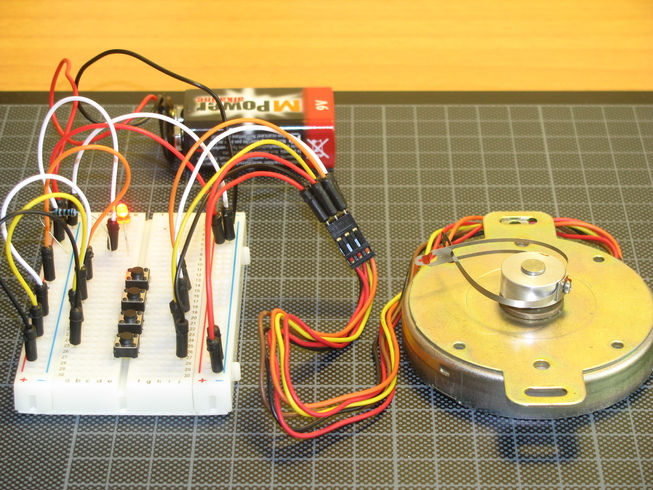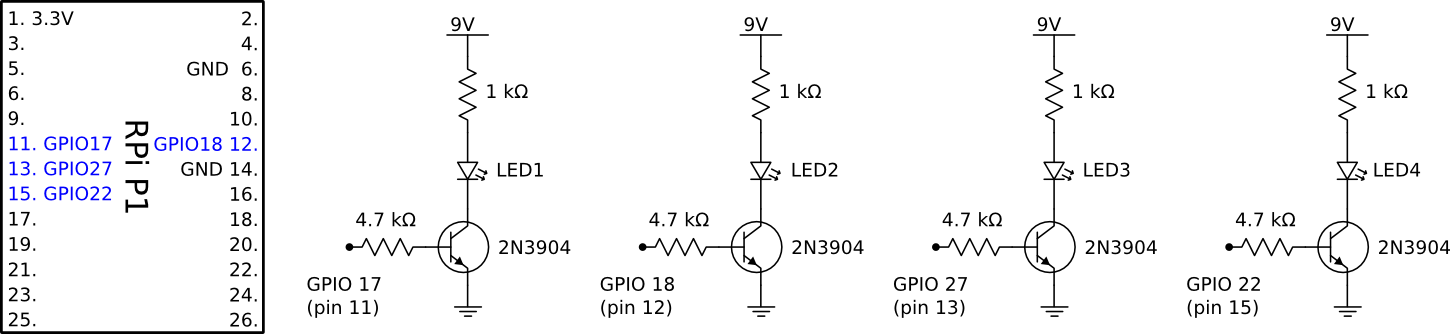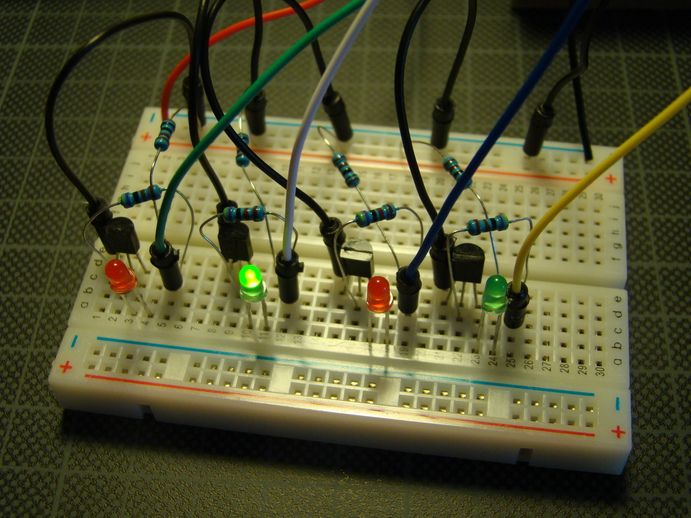Prototypes for stepper motor control

I have a unipolar stepper motor I want to control with my Raspberry Pi. First I needed to figure out the motor coils’ wiring, then I wrote a small test script to provide the correct sequence on the GPIO ports.

WiringA 9V battery, an indicator led to show if the power is on, and four switches. When a switch is pressed one phase (half coil) is energized. For some unknown reason I managed to get the wiring right for the first time, so pressing the switches one after the other, from top to bottom, made the motor turn one step.

The 6 wires of this motor (Japan Servo KP68P2-406, 12V, 33 Ω/phase, 1.8 deg/step) are the following:

• 2 red (center taps)
• orange (phase 1)
• brown (phase 2)
• yellow (phase 3)
• black (phase 4)

Sequence test

I wrote a small test script to output the step sequence on the Pi’s GPIO ports. It has a lot of cool features:

\$ sudo python stepper_seq.py -h  # help
\$ sudo python stepper_seq.py  # turns the motor indefinitely, Control-C terminates
\$ sudo python stepper_seq.py -s half -r -n 4  # 4 times the half-step sequence, reversed
\$ sudo python stepper_seq.py -s kitt  # nothing to do with stepper motors ;)

And the code of stepper_seq.py:

#!/usr/bin/env python
# stepper_seq.py - Stepper motor sequence test
# Copyright (C) 2013 David Wagner
from itertools import chain, cycle, repeat
import argparse
import RPi.GPIO as GPIO
import signal
import sys
import time

sequences = {# Wave Drive, One-Phase
'one': [[True, False, False, False],
[False, True, False, False],
[False, False, True, False],
[False, False, False, True]],
# Hi-Torque, Two-Phase
'two': [[True, True, False, False],
[False, True, True, False],
[False, False, True, True],
[True, False, False, True]],
# Half-Step
'half': [[True, False, False, False],
[True, True, False, False],
[False, True, False, False],
[False, True, True, False],
[False, False, True, False],
[False, False, True, True],
[False, False, False, True],
[True, False, False, True]]}

sequences.update({'kitt': (sequences['one'][:-1] +
list(reversed(sequences['one'])))})

parser = argparse.ArgumentParser(description='Stepper motor sequence test.')
default=[11, 12, 13, 15],
help='GPIO pins to activate (numbering by BOARD)')
choices=sorted(sequences.keys()),
help='Coil activation sequence.')
help='Delay in seconds.')
help='Reverse direction.')
help='Number of complete cycles.')

args = parser.parse_args()

def init_board():
GPIO.setmode(GPIO.BOARD)
for pin in args.pins:
GPIO.setup(pin, GPIO.OUT)

def init_pins():
for pin in args.pins:
GPIO.output(pin, False)

def signal_handler(signal, frame):
print "Interrupted. Exiting..."
init_pins()
sys.exit(0)

def ncycles(iterable, n):
"Returns the sequence elements n times"
return chain.from_iterable(repeat(tuple(iterable), n))

def step(num=0):
pin_order = list(reversed(args.pins)) if args.reverse else args.pins
rep = cycle if num == 0 else lambda it: ncycles(it, num)
for seq in rep(sequences[args.seq]):
for pin, status in zip(pin_order, seq):
GPIO.output(pin, status)
time.sleep(args.delay)

def main():
signal.signal(signal.SIGINT, signal_handler)
init_board()
init_pins()
step(args.num)

if __name__ == '__main__':
main()

Sequence test hardware

I put together a circuit to test the script above. It contains four LEDs, each connected to one of the Pi’s GPIO ports through a transistor. The LED’s could be directly connected to the IO ports, but in this project I wanted to refresh how to use a transistor as a switch so that the LEDs can be powered from an external power supply.And on the breadboard it looks like this: